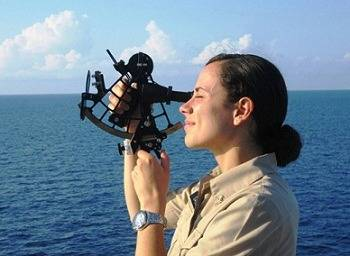# How Is Trigonometry Used In Oceanography?

Trigonometry is used in oceanography for calculating the intensity and height of tides in oceans.Using trigonometry in ratios, it is possible to determine the distance between yourself and the object so Oceanographers use trigonometry to measure distance.

For example, if the oceanographer was tracking a sea creature and they needed to know how far away they were from the creature, (as far away as possible if you ask me!) they would use trigonometry to find out the distance from them and the creature.

A semidiurnal tide is a body of water,  like the Atlantic Ocean, that has two high tides and two low tides each and every day because tides behave the same way in a given period. An Oceanographer would use trignonometry to plot a graph showing the size of the tide at each time of day - high and low tide

thanked the writer.

hello

thanked the writer.